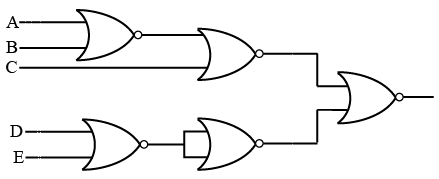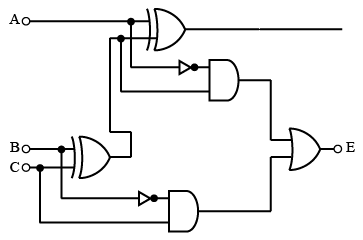# ISRO Scientist or Engineer Computer Science 2007

Instructions

For the following questions answer them individually

Question 1

# The Boolean expression $$Y =(A +\overline{B}+ \overline{A}B)\overline{C}$$ is given byQuestion 2

# The circuit shown in the following Figure realizes the functionQuestion 3

# The circuit shown in the given Figure is aQuestion 4

# When two numbers are added in excess-3 code and the sum is less than 9,then in order to get the correct answerit is necessary toQuestion 5

# The characteristic equation of an SR flip-flop is given byQuestion 6

# Agraph with "n" vertices and n-1 edges thatis not a tree,isQuestion 7

# If a graph requires k different colours for its proper colouring, then the chromatic numberof the graphisQuestion 8Question 9

# Eigen vectors of $$\begin{bmatrix}1 & \cos \theta \\\cos \theta &1 \end{bmatrix}$$ areQuestion 10

# The term "aging"refers toOR